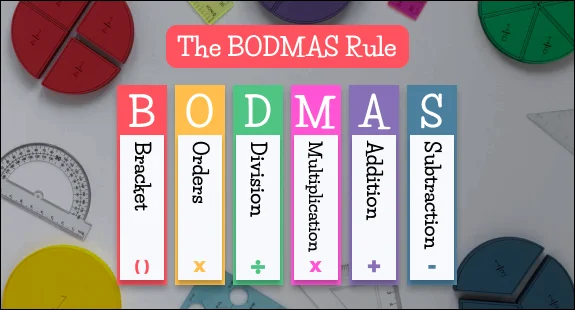# Maths blog - BODMAS Rule

I am sure you have heard the abbreviation “BODMAS” at least once in your life. I am also certain that it happened to be in a mathematics lecture. While to most of us, mathematics is a subject of fear, there are some formulae at our disposal that make it comparatively easier. One such example is the – BODMAS Rule.

BODMAS has proven to be very handy while solving even the most complex mathematical expressions. As someone who has struggled with numbers, I found BODMAS to be a boon and it was responsible for me scoring decent marks in my school life. So, if you are in the same boat, don’t worry I’ve got you covered! And if you’re someone who takes pride in their mathematical skills, you should still read till the end because brushing up your skills is never a bad idea!

So, let’s Begin!

The rule or order that we use to simplify expressions in math is called BODMAS rule. Let’s see what it stands for.

Very simply way to remember the BODMAS rule!

B — Brackets

O — Of (orders: Powers and radicals)

D — Division

M — Multiplication

S — SubtractionImportant Notes:

1. In a particular simplification, if you have both multiplication and division, do the operations one by one in the order from left to right.

2. Division does not always come before multiplication. We must do one by one in the order from left to right.

3. In a particular simplification, if you have both addition and subtraction, do the operations one by one in the order from left to right

Examples:

12 ÷ 3 x 5 = 4 x 5 = 20

13 – 5 + 9 = 8 + 9 = 17

In the above simplification, we have both division and multiplication. From left to right, we have division first and multiplication next. So, we do division first and multiplication next.

Solved Problems – BODMAS RULE

Problem 1:

Evaluate:  6 + 7 x 8

Solution:

 Evaluation = 6 + 7 x 8= 6 + 56= 62 Operation MultiplicationAdditionResult

Problem 2:

Evaluate: 102 – 16 ÷ 8

Solution:

 Evaluation = 102 – 16 ÷ 8= 100 – 16 ÷ 8= 100 – 2= 98 Operation PowerDivisionSubtractionResult

Problem 3:

Evaluate: (25 + 11) x 2

Solution:

 Evaluation = (25 + 11) x 2= 36 x 2= 72 Operation BracketMultiplicationResult

Problem 4:

Evaluate: 3 + 6 x (5 + 4) ÷ 3 -7

Solution:

 Evaluation = 3 + 6 x (5 + 4) ÷ 3 -7= 3 + 6 x 9 ÷ 3 -7= 3 + 54 ÷ 3 -7= 3 + 18 -7= 21 – 7= 14 Operation BracketMultiplicationDivisionAdditionSubtractionResult

Problem 5:

Evaluate: 56 – 2(20 + 12 ÷ 4 x 3 – 2 x 2) + 10

Solution:

 Evaluation = 56 – 2(20 + 12 ÷ 4 x 3 – 2 x 2) + 10= 56 – 2(20 + 12 ÷ 4 x 3 – 2 x 2) + 10= 56 – 2(20 + 3 x 3 – 2 x 2) + 10= 56 – 2(20 + 9 – 4) + 10= 56 – 2(29 – 4) + 10= 56 – 2(25) + 10= 56 – 50 + 10= 6 + 10= 16 Operation BracketDivisionMultiplicationAdditionSubtractionMultiplicationSubtractionAdditionResult

Problem 6:

Evaluate: 6 + [(16 – 4) ÷ (22 + 2)] – 2

Solution:

 Evaluation = 6 + [(16 – 4) ÷ (22 + 2)] – 2= 6 + [12 ÷ (22 + 2)] – 2= 6 + [12 ÷ (4 + 2)] – 2= 6 + [12 ÷ 6] – 2= 6 + 2 – 2= 8 – 2= 6 Operation Square BracketPowerBracketSquare BracketAdditionSubtractionResult

Problem 7:

Evaluate: (96 ÷ 12) + 14 x (12 + 8) ÷ 2

Solution:

 Evaluation = (96 ÷ 12) + 14 x (12 + 8) ÷ 2= 8 + 14 x 20 ÷ 2= 8 + 280 ÷ 2= 8 + 140= 148 Operation BracketMultiplicationDivisionAdditionResult

Problem 8:

Evaluate: (93 + 15) ÷ (3 x 4) – 24 + 8

Solution:

 Evaluation = (93 + 15) ÷ (3 x 4) – 24 + 8= 108 ÷ 12 – 24 + 8= 9 – 24 + 8= -15 + 8= -7 Operation BracketDivisionSubtractionSubtractionResult

Problem 9:

Evaluate: 55 ÷ 11 + (18 – 6) x 9

Solution:

 Evaluation = 55 ÷ 11 + (18 – 6) x 9= 55 ÷ 11 + 12 x 9= 5 + 12 x 9= 5 + 108= 113 Operation BracketDivisionMultiplicationAdditionResult

Problem 10:

Evaluate: (7 + 18) x 3 ÷ (2 + 13) – 28

Solution:

 Evaluation = (7 + 18) x 3 ÷ (2 + 13) – 28= 25 x 3 ÷ 15 – 28= 75 ÷ 15 – 28= 5 – 28= -23 Operation BracketMultiplicationDivisionSubtractionResult

Hope these examples and the explanation regarding the BODMAS rule was beneficial to you.

For much such informative yet fun blogs — Top 10 Facts about puzzles